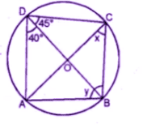Guru

# Question 4. (a) In the figure (i) given below, calculate the values of x and y.

• 1

This is an important and exam oriented question from Chapter name- circles
Topic – Angle properties of circles
Chapter number- 15

In this question we have been given the figure of circle with certain information and we have to find the value of x in this figure.
ICSE Avichal publication
Understanding ICSE Mathematics
Question4 (a)

Share

1. Solution:∠B + ∠D = 1800

Y + 40+ 45= 180o

(y + 85= 180o)

Y = 180– 85o = 95o